# GLM in R: Generalized Linear Model with Example

## What is Logistic regression?

Logistic regression is used to predict a class, i.e., a probability. Logistic regression can predict a binary outcome accurately.

Imagine you want to predict whether a loan is denied/accepted based on many attributes. The logistic regression is of the form 0/1. y = 0 if a loan is rejected, y = 1 if accepted.

A logistic regression model differs from linear regression model in two ways.

• First of all, the logistic regression accepts only dichotomous (binary) input as a dependent variable (i.e., a vector of 0 and 1).
• Secondly, the outcome is measured by the following probabilistic link function called sigmoid due to its S-shaped.: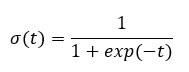The output of the function is always between 0 and 1. Check Image below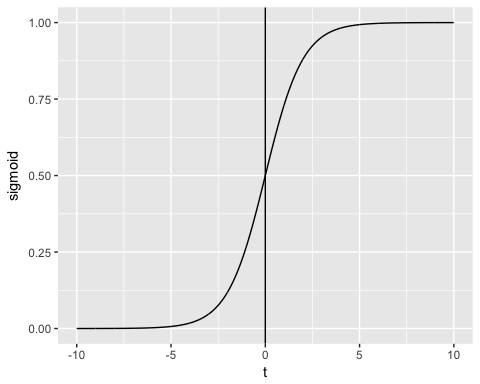The sigmoid function returns values from 0 to 1. For the classification task, we need a discrete output of 0 or 1.

To convert a continuous flow into discrete value, we can set a decision bound at 0.5. All values above this threshold are classified as 1

Learn Java Programming with Beginners Tutorial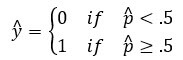## How to create Generalized Liner Model (GLM)

Let’s use the adult data set to illustrate Logistic regression. The “adult” is a great dataset for the classification task. The objective is to predict whether the annual income in dollar of an individual will exceed 50.000. The dataset contains 46,033 observations and ten features:

• age: age of the individual. Numeric
• education: Educational level of the individual. Factor.
• marital.status: Marital status of the individual. Factor i.e. Never-married, Married-civ-spouse, …
• gender: Gender of the individual. Factor, i.e. Male or Female
• income: Target variable. Income above or below 50K. Factor i.e. >50K, <=50K

amongst others

Output:

Observations: 48,842 Variables: 10 \$ x 1, 2, 3, 4, 5, 6, 7, 8, 9, 10, 11, 12, 13, 14, 15,… \$ age 25, 38, 28, 44, 18, 34, 29, 63, 24, 55, 65, 36, 26… \$ workclass Private, Private, Local-gov, Private, ?, Private,… \$ education 11th, HS-grad, Assoc-acdm, Some-college, Some-col… \$ educational.num 7, 9, 12, 10, 10, 6, 9, 15, 10, 4, 9, 13, 9, 9, 9,… \$ marital.status Never-married, Married-civ-spouse, Married-civ-sp… \$ race Black, White, White, Black, White, White, Black, … \$ gender Male, Male, Male, Male, Female, Male, Male, Male,… \$ hours.per.week 40, 50, 40, 40, 30, 30, 40, 32, 40, 10, 40, 40, 39… \$ income <=50K, <=50K, >50K, >50K, <=50K, <=50K, <=50K, >5…

We will proceed as follow:

• Step 1: Check continuous variables
• Step 2: Check factor variables
• Step 3: Feature engineering
• Step 4: Summary statistic
• Step 5: Train/test set
• Step 6: Build the model
• Step 7: Assess the performance of the model
• step 8: Improve the model

Your task is to predict which individual will have a revenue higher than 50K.

In this tutorial, each step will be detailed to perform an analysis on a real dataset.

### Step 1) Check continuous variables

In the first step, you can see the distribution of the continuous variables.

Code Explanation

• continuous <- select_if(data_adult, is.numeric): Use the function select_if() from the dplyr library to select only the numerical columns
• summary(continuous): Print the summary statistic

Output:

## X age educational.num hours.per.week ## Min. : 1 Min. :17.00 Min. : 1.00 Min. : 1.00 ## 1st Qu.:11509 1st Qu.:28.00 1st Qu.: 9.00 1st Qu.:40.00 ## Median :23017 Median :37.00 Median :10.00 Median :40.00 ## Mean :23017 Mean :38.56 Mean :10.13 Mean :40.95 ## 3rd Qu.:34525 3rd Qu.:47.00 3rd Qu.:13.00 3rd Qu.:45.00 ## Max. :46033 Max. :90.00 Max. :16.00 Max. :99.00

From the above table, you can see that the data have totally different scales and hours.per.weeks has large outliers (.i.e. look at the last quartile and maximum value).

You can deal with it following two steps:

• 1: Plot the distribution of hours.per.week
• 2: Standardize the continuous variables
1. Plot the distribution

Let’s look closer at the distribution of hours.per.week

# Histogram with kernel density curve library(ggplot2) ggplot(continuous, aes(x = hours.per.week)) + geom_density(alpha = .2, fill = “#FF6666”)

Output: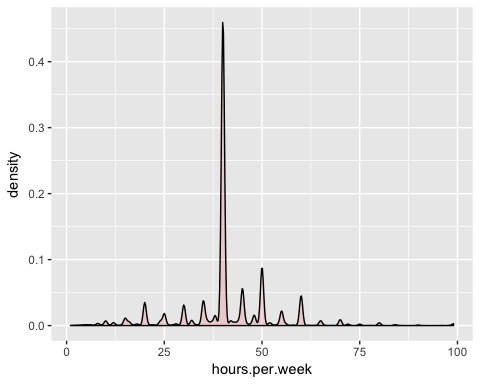The variable has lots of outliers and not well-defined distribution. You can partially tackle this problem by deleting the top 0.01 percent of the hours per week.

Basic syntax of quantile:

quantile(variable, percentile) arguments: -variable: Select the variable in the data frame to compute the percentile -percentile: Can be a single value between 0 and 1 or multiple value. If multiple, use this format: `c(A,B,C, ...) - `A`,`B`,`C`and`…` are all integer from 0 to 1.

We compute the top 2 percent percentile

Code Explanation

• quantile(data_adult\$hours.per.week, .99): Compute the value of the 99 percent of the working time

Output:

## 99% ## 80

98 percent of the population works under 80 hours per week.

You can drop the observations above this threshold. You use the filter from the dplyr library.

Output:

##  45537 10

1. Standardize the continuous variables

You can standardize each column to improve the performance because your data do not have the same scale. You can use the function mutate_if from the dplyr library. The basic syntax is:

mutate_if(df, condition, funs(function)) arguments: -`df`: Data frame used to compute the function - `condition`: Statement used. Do not use parenthesis - funs(function): Return the function to apply. Do not use parenthesis for the function

You can standardize the numeric columns as follow:

Code Explanation

• mutate_if(is.numeric, funs(scale)): The condition is only numeric column and the function is scale

Output:

## X age workclass education educational.num ## 1 -1.732680 -1.02325949 Private 11th -1.22106443 ## 2 -1.732605 -0.03969284 Private HS-grad -0.43998868 ## 3 -1.732530 -0.79628257 Local-gov Assoc-acdm 0.73162494 ## 4 -1.732455 0.41426100 Private Some-college -0.04945081 ## 5 -1.732379 -0.34232873 Private 10th -1.61160231 ## 6 -1.732304 1.85178149 Self-emp-not-inc Prof-school 1.90323857 ## marital.status race gender hours.per.week income ## 1 Never-married Black Male -0.03995944 <=50K ## 2 Married-civ-spouse White Male 0.86863037 <=50K ## 3 Married-civ-spouse White Male -0.03995944 >50K ## 4 Married-civ-spouse Black Male -0.03995944 >50K ## 5 Never-married White Male -0.94854924 <=50K ## 6 Married-civ-spouse White Male -0.76683128 >50K

### Step 2) Check factor variables

This step has two objectives:

• Check the level in each categorical column
• Define new levels

We will divide this step into three parts:

• Select the categorical columns
• Store the bar chart of each column in a list
• Print the graphs

We can select the factor columns with the code below:

# Select categorical column factor <- data.frame(select_if(data_adult_rescale, is.factor)) ncol(factor)

Code Explanation

• data.frame(select_if(data_adult, is.factor)): We store the factor columns in factor in a data frame type. The library ggplot2 requires a data frame object.

Output:

##  6

The dataset contains 6 categorical variables

The second step is more skilled. You want to plot a bar chart for each column in the data frame factor. It is more convenient to automatize the process, especially in situation there are lots of columns.

library(ggplot2) # Create graph for each column graph <- lapply(names(factor), function(x) ggplot(factor, aes(get(x))) + geom_bar() + theme(axis.text.x = element_text(angle = 90)))

Code Explanation

• lapply(): Use the function lapply() to pass a function in all the columns of the dataset. You store the output in a list
• function(x): The function will be processed for each x. Here x is the columns
• ggplot(factor, aes(get(x))) + geom_bar()+ theme(axis.text.x = element_text(angle = 90)): Create a bar char chart for each x element. Note, to return x as a column, you need to include it inside the get()

The last step is relatively easy. You want to print the 6 graphs.

# Print the graph graph

Output:

## []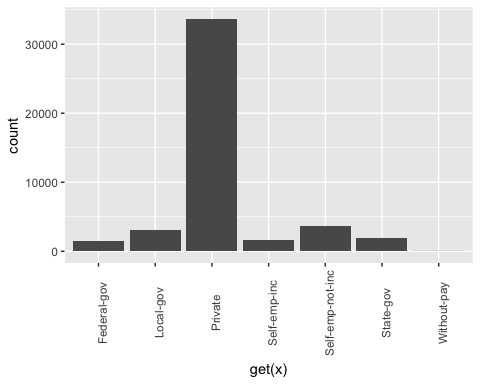## ## []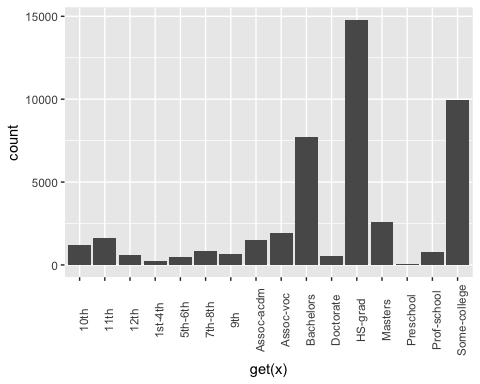## ## []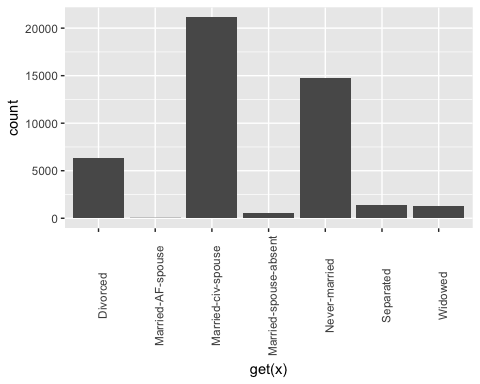## ## []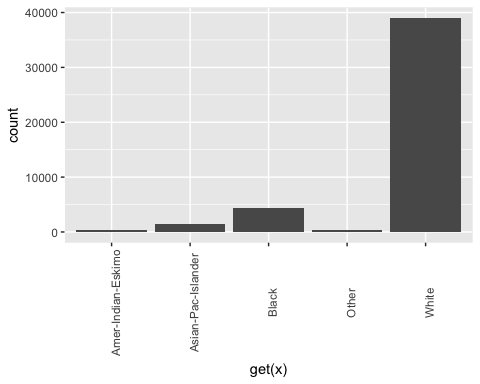## ## []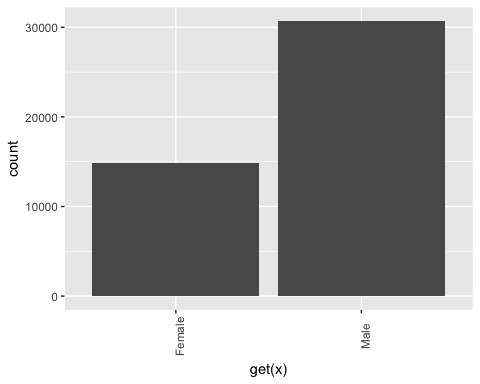## ## []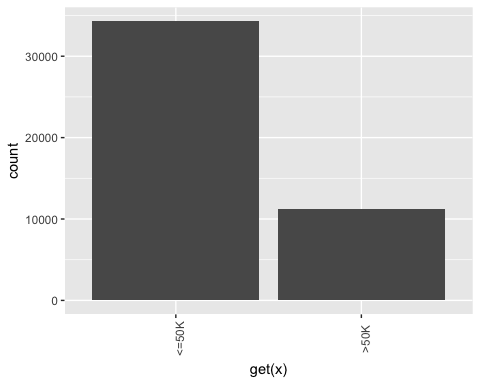Note: Use the next button to navigate to the next graph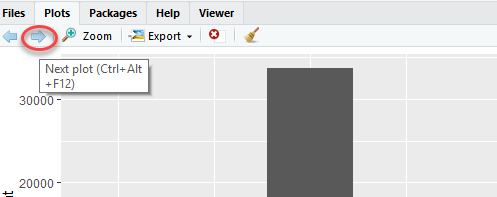### Recast education

From the graph above, you can see that the variable education has 16 levels. This is substantial, and some levels have a relatively low number of observations. If you want to improve the amount of information you can get from this variable, you can recast it into higher level. Namely, you create larger groups with similar level of education. For instance, low level of education will be converted in dropout. Higher levels of education will be changed to master.

Here is the detail:

Old level New level
Preschool dropout
10th Dropout
11th Dropout
12th Dropout
1st-4th Dropout
5th-6th Dropout
7th-8th Dropout
9th Dropout
Some-college Community
Assoc-acdm Community
Assoc-voc Community
Bachelors Bachelors
Masters Masters
Prof-school Masters
Doctorate PhD

recast_data <- data_adult_rescale % > % select(-X) % > % mutate(education = factor(ifelse(education == “Preschool” | education == “10th” | education == “11th” | education == “12th” | education == “1st-4th” | education == “5th-6th” | education == “7th-8th” | education == “9th”, “dropout”, ifelse(education == “HS-grad”, “HighGrad”, ifelse(education == “Some-college” | education == “Assoc-acdm” | education == “Assoc-voc”, “Community”, ifelse(education == “Bachelors”, “Bachelors”, ifelse(education == “Masters” | education == “Prof-school”, “Master”, “PhD”)))))))

Code Explanation

• We use the verb mutate from dplyr library. We change the values of education with the statement ifelse

In the table below, you create a summary statistic to see, on average, how many years of education (z-value) it takes to reach the Bachelor, Master or PhD.

recast_data % > % group_by(education) % > % summarize(average_educ_year = mean(educational.num), count = n()) % > % arrange(average_educ_year)

Output:

## # A tibble: 6 x 3 ## education average_educ_year count ## ## 1 dropout -1.76147258 5712 ## 2 HighGrad -0.43998868 14803 ## 3 Community 0.09561361 13407 ## 4 Bachelors 1.12216282 7720 ## 5 Master 1.60337381 3338 ## 6 PhD 2.29377644 557

### Recast Marital-status

It is also possible to create lower levels for the marital status. In the following code you change the level as follow:

Old level New level
Never-married Not-married
Married-spouse-absent Not-married
Married-AF-spouse Married
Married-civ-spouse
Separated Separated
Divorced
Widows Widow

# Change level marry recast_data <- recast_data % > % mutate(marital.status = factor(ifelse(marital.status == “Never-married” | marital.status == “Married-spouse-absent”, “Not_married”, ifelse(marital.status == “Married-AF-spouse” | marital.status == “Married-civ-spouse”, “Married”, ifelse(marital.status == “Separated” | marital.status == “Divorced”, “Separated”, “Widow”)))))

You can check the number of individuals within each group.

table(recast_data\$marital.status)

Output:

## ## Married Not_married Separated Widow ## 21165 15359 7727 1286

### Step 4) Summary Statistic

It is time to check some statistics about our target variables. In the graph below, you count the percentage of individuals earning more than 50k given their gender.

# Plot gender income ggplot(recast_data, aes(x = gender, fill = income)) + geom_bar(position = “fill”) + theme_classic()

Output:Next, check if the origin of the individual affects their earning.

# Plot origin income ggplot(recast_data, aes(x = race, fill = income)) + geom_bar(position = “fill”) + theme_classic() + theme(axis.text.x = element_text(angle = 90))

Output: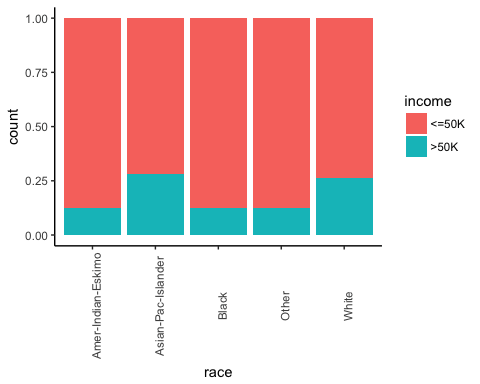The number of hours work by gender.

# box plot gender working time ggplot(recast_data, aes(x = gender, y = hours.per.week)) + geom_boxplot() + stat_summary(fun.y = mean, geom = “point”, size = 3, color = “steelblue”) + theme_classic()

Output:The box plot confirms that the distribution of working time fits different groups. In the box plot, both genders do not have homogeneous observations.

You can check the density of the weekly working time by type of education. The distributions have many distinct picks. It can probably be explained by the type of contract in the US.

# Plot distribution working time by education ggplot(recast_data, aes(x = hours.per.week)) + geom_density(aes(color = education), alpha = 0.5) + theme_classic()

Code Explanation

• ggplot(recast_data, aes( x= hours.per.week)): A density plot only requires one variable
• geom_density(aes(color = education), alpha =0.5): The geometric object to control the density

Output: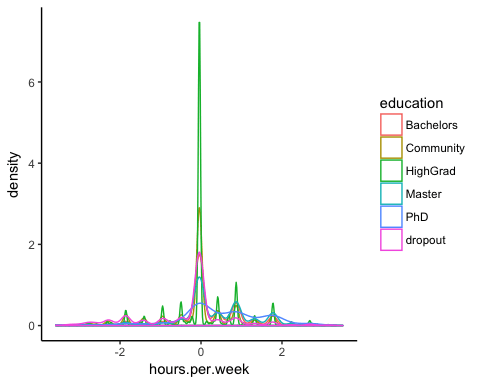To confirm your thoughts, you can perform a one-way ANOVA test:

anova <- aov(hours.per.week~education, recast_data) summary(anova)

Output:

## Df Sum Sq Mean Sq F value Pr(>F) ## education 5 1552 310.31 321.2 <2e-16 *** ## Residuals 45531 43984 0.97 ## — ## Signif. codes: 0 ‘’ 0.001 '’ 0.01 '’ 0.05 ‘.’ 0.1 ’ ’ 1

The ANOVA test confirms the difference in average between groups.

### Non-linearity

Before you run the model, you can see if the number of hours worked is related to age.

library(ggplot2) ggplot(recast_data, aes(x = age, y = hours.per.week)) + geom_point(aes(color = income), size = 0.5) + stat_smooth(method = ‘lm’, formula = y~poly(x, 2), se = TRUE, aes(color = income)) + theme_classic()

Code Explanation

• ggplot(recast_data, aes(x = age, y = hours.per.week)): Set the aesthetic of the graph
• geom_point(aes(color= income), size =0.5): Construct the dot plot
• stat_smooth(): Add the trend line with the following arguments:
• method=‘lm’: Plot the fitted value if the linear regression
• formula = y~poly(x,2): Fit a polynomial regression
• se = TRUE: Add the standard error
• aes(color= income): Break the model by income

Output: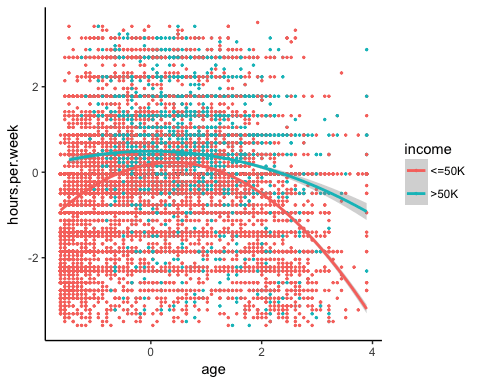In a nutshell, you can test interaction terms in the model to pick up the non-linearity effect between the weekly working time and other features. It is important to detect under which condition the working time differs.

### Correlation

The next check is to visualize the correlation between the variables. You convert the factor level type to numeric so that you can plot a heat map containing the coefficient of correlation computed with the Spearman method.

library(GGally) # Convert data to numeric corr <- data.frame(lapply(recast_data, as.integer)) # Plot the graphggcorr(corr, method = c(“pairwise”, “spearman”), nbreaks = 6, hjust = 0.8, label = TRUE, label_size = 3, color = “grey50”)

Code Explanation

• data.frame(lapply(recast_data,as.integer)): Convert data to numeric
• ggcorr() plot the heat map with the following arguments:
• method: Method to compute the correlation
• nbreaks = 6: Number of break
• hjust = 0.8: Control position of the variable name in the plot
• label = TRUE: Add labels in the center of the windows
• label_size = 3: Size labels
• color = “grey50”): Color of the label

Output: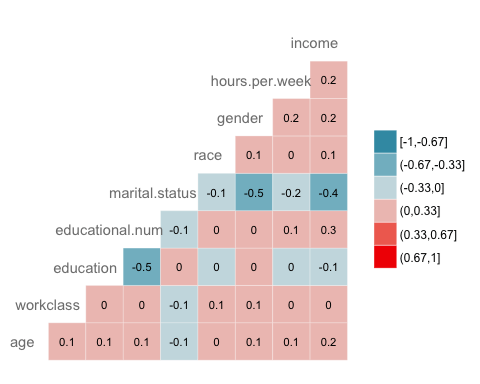### Step 5) Train/test set

Any supervised machine learning task require to split the data between a train set and a test set. You can use the “function” you created in the other supervised learning tutorials to create a train/test set.

set.seed(1234) create_train_test <- function(data, size = 0.8, train = TRUE) { n_row = nrow(data) total_row = size * n_row train_sample <- 1: total_row if (train == TRUE) { return (data[train_sample, ]) } else { return (data[-train_sample, ]) } } data_train <- create_train_test(recast_data, 0.8, train = TRUE) data_test <- create_train_test(recast_data, 0.8, train = FALSE) dim(data_train)

Output:

dim(data_test)

Output:

##  9108 9

### Step 6) Build the model

To see how the algorithm performs, you use the glm() package. The Generalized Linear Model is a collection of models. The basic syntax is:

glm(formula, data=data, family=linkfunction() Argument: - formula: Equation used to fit the model- data: dataset used - Family: - binomial: (link = “logit”) - gaussian: (link = “identity”) - Gamma: (link = “inverse”) - inverse.gaussian: (link = “1/mu^2”) - poisson: (link = “log”) - quasi: (link = “identity”, variance = “constant”) - quasibinomial: (link = “logit”) - quasipoisson: (link = “log”)

You are ready to estimate the logistic model to split the income level between a set of features.

formula <- income~. logit <- glm(formula, data = data_train, family = ‘binomial’) summary(logit)

Code Explanation

• formula <- income ~ .: Create the model to fit
• logit <- glm(formula, data = data_train, family = ‘binomial’): Fit a logistic model (family = ‘binomial’) with the data_train data.
• summary(logit): Print the summary of the model

Output:

## ## Call: ## glm(formula = formula, family = “binomial”, data = data_train) ## ## Deviance Residuals: ## Min 1Q Median 3Q Max ## -2.6456 -0.5858 -0.2609 -0.0651 3.1982 ## ## Coefficients: ## Estimate Std. Error z value Pr(>|z|) ## (Intercept) 0.07882 0.21726 0.363 0.71675 ## age 0.41119 0.01857 22.146 < 2e-16 *** ## workclassLocal-gov -0.64018 0.09396 -6.813 9.54e-12 *** ## workclassPrivate -0.53542 0.07886 -6.789 1.13e-11 *** ## workclassSelf-emp-inc -0.07733 0.10350 -0.747 0.45499 ## workclassSelf-emp-not-inc -1.09052 0.09140 -11.931 < 2e-16 *** ## workclassState-gov -0.80562 0.10617 -7.588 3.25e-14 *** ## workclassWithout-pay -1.09765 0.86787 -1.265 0.20596 ## educationCommunity -0.44436 0.08267 -5.375 7.66e-08 *** ## educationHighGrad -0.67613 0.11827 -5.717 1.08e-08 *** ## educationMaster 0.35651 0.06780 5.258 1.46e-07 *** ## educationPhD 0.46995 0.15772 2.980 0.00289 ** ## educationdropout -1.04974 0.21280 -4.933 8.10e-07 *** ## educational.num 0.56908 0.07063 8.057 7.84e-16 *** ## marital.statusNot_married -2.50346 0.05113 -48.966 < 2e-16 *** ## marital.statusSeparated -2.16177 0.05425 -39.846 < 2e-16 *** ## marital.statusWidow -2.22707 0.12522 -17.785 < 2e-16 *** ## raceAsian-Pac-Islander 0.08359 0.20344 0.411 0.68117 ## raceBlack 0.07188 0.19330 0.372 0.71001 ## raceOther 0.01370 0.27695 0.049 0.96054 ## raceWhite 0.34830 0.18441 1.889 0.05894 . ## genderMale 0.08596 0.04289 2.004 0.04506 * ## hours.per.week 0.41942 0.01748 23.998 < 2e-16 *** ## —## Signif. codes: 0 ‘’ 0.001 '’ 0.01 '’ 0.05 ‘.’ 0.1 ’ ’ 1 ## ## (Dispersion parameter for binomial family taken to be 1) ## ## Null deviance: 40601 on 36428 degrees of freedom ## Residual deviance: 27041 on 36406 degrees of freedom ## AIC: 27087 ## ## Number of Fisher Scoring iterations: 6

The summary of our model reveals interesting information. The performance of a logistic regression is evaluated with specific key metrics.

• AIC (Akaike Information Criteria): This is the equivalent of R2 in logistic regression. It measures the fit when a penalty is applied to the number of parameters. Smaller AIC values indicate the model is closer to the truth.
• Null deviance: Fits the model only with the intercept. The degree of freedom is n-1. We can interpret it as a Chi-square value (fitted value different from the actual value hypothesis testing).
• Residual Deviance: Model with all the variables. It is also interpreted as a Chi-square hypothesis testing.
• Number of Fisher Scoring iterations: Number of iterations before converging.

The output of the glm() function is stored in a list. The code below shows all the items available in the logit variable we constructed to evaluate the logistic regression.

# The list is very long, print only the first three elements

lapply(logit, class)[1:3]

Output:

## \$coefficients ##  “numeric” ## ## \$residuals ##  “numeric” ## ## \$fitted.values ##  “numeric”

Each value can be extracted with the \$ sign follow by the name of the metrics. For instance, you stored the model as logit. To extract the AIC criteria, you use:

logit\$aic

Output:

##  27086.65

### Step 7) Assess the performance of the model

##### Confusion Matrix

The confusion matrix is a better choice to evaluate the classification performance compared with the different metrics you saw before. The general idea is to count the number of times True instances are classified are False.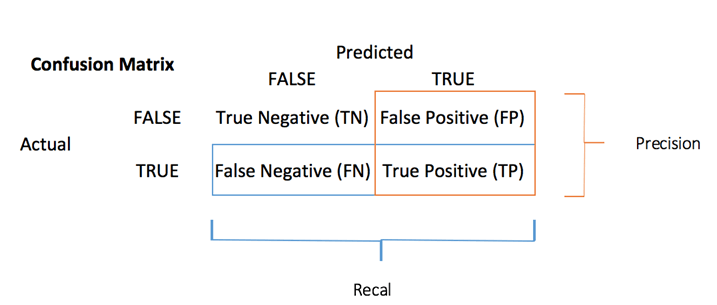To compute the confusion matrix, you first need to have a set of predictions so that they can be compared to the actual targets.

predict <- predict(logit, data_test, type = ‘response’) # confusion matrix table_mat <- table(data_test\$income, predict > 0.5) table_mat

Code Explanation

• predict(logit,data_test, type = ‘response’): Compute the prediction on the test set. Set type = ‘response’ to compute the response probability.
• table(data_test\$income, predict > 0.5): Compute the confusion matrix. predict > 0.5 means it returns 1 if the predicted probabilities are above 0.5, else 0.

Output:

## ## FALSE TRUE ## <=50K 6310 495 ## >50K 1074 1229

Each row in a confusion matrix represents an actual target, while each column represents a predicted target. The first row of this matrix considers the income lower than 50k (the False class): 6241 were correctly classified as individuals with income lower than 50k ( True negative ), while the remaining one was wrongly classified as above 50k ( False positive ). The second row considers the income above 50k, the positive class were 1229 ( True positive ), while the True negative was 1074.

You can calculate the model accuracy by summing the true positive + true negative over the total observation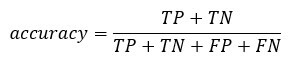accuracy_Test <- sum(diag(table_mat)) / sum(table_mat) accuracy_Test

Code Explanation

• sum(diag(table_mat)): Sum of the diagonal
• sum(table_mat): Sum of the matrix.

Output:

##  0.8277339

The model appears to suffer from one problem, it overestimates the number of false negatives. This is called the accuracy test paradox . We stated that the accuracy is the ratio of correct predictions to the total number of cases. We can have relatively high accuracy but a useless model. It happens when there is a dominant class. If you look back at the confusion matrix, you can see most of the cases are classified as true negative. Imagine now, the model classified all the classes as negative (i.e. lower than 50k). You would have an accuracy of 75 percent (6718/6718+2257). Your model performs better but struggles to distinguish the true positive with the true negative.

In such situation, it is preferable to have a more concise metric. We can look at:

• Precision=TP/(TP+FP)
• Recall=TP/(TP+FN)

### Precision vs Recall

Precision looks at the accuracy of the positive prediction. Recall is the ratio of positive instances that are correctly detected by the classifier;

You can construct two functions to compute these two metrics

1. Construct precision

precision <- function(matrix) { # True positive tp <- matrix[2, 2] # false positive fp <- matrix[1, 2] return (tp / (tp + fp)) }

Code Explanation

• mat[1,1]: Return the first cell of the first column of the data frame, i.e. the true positive
• mat[1,2]; Return the first cell of the second column of the data frame, i.e. the false positive

recall <- function(matrix) { # true positive tp <- matrix[2, 2]# false positive fn <- matrix[2, 1] return (tp / (tp + fn)) }

Code Explanation

• mat[1,1]: Return the first cell of the first column of the data frame, i.e. the true positive
• mat[2,1]; Return the second cell of the first column of the data frame, i.e. the false negative

prec <- precision(table_mat) prec rec <- recall(table_mat) rec

Output:

##  0.712877 ##  0.5336518

When the model says it is an individual above 50k, it is correct in only 54 percent of the case, and can claim individuals above 50k in 72 percent of the case.

You can create the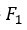score based on the precision and recall. Theis a harmonic mean of these two metrics, meaning it gives more weight to the lower values.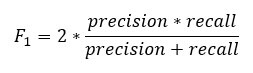f1 <- 2 * ((prec * rec) / (prec + rec)) f1

Output:

##  0.6103799

It is impossible to have both a high precision and high recall.

If we increase the precision, the correct individual will be better predicted, but we would miss lots of them (lower recall). In some situation, we prefer higher precision than recall. There is a concave relationship between precision and recall.

• Imagine, you need to predict if a patient has a disease. You want to be as precise as possible.
• If you need to detect potential fraudulent people in the street through facial recognition, it would be better to catch many people labeled as fraudulent even though the precision is low. The police will be able to release the non-fraudulent individual.

### The ROC curve

The Receiver Operating Characteristic curve is another common tool used with binary classification. It is very similar to the precision/recall curve, but instead of plotting precision versus recall, the ROC curve shows the true positive rate (i.e., recall) against the false positive rate. The false positive rate is the ratio of negative instances that are incorrectly classified as positive. It is equal to one minus the true negative rate. The true negative rate is also called specificity . Hence the ROC curve plots sensitivity (recall) versus 1-specificity

To plot the ROC curve, we need to install a library called RORC. We can find in the conda library. You can type the code:

conda install -c r r-rocr --yes

We can plot the ROC with the prediction() and performance() functions.

library(ROCR) ROCRpred <- prediction(predict, data_test\$income) ROCRperf <- performance(ROCRpred, ‘tpr’, ‘fpr’) plot(ROCRperf, colorize = TRUE, text.adj = c(-0.2, 1.7))

Code Explanation

• prediction(predict, data_test\$income): The ROCR library needs to create a prediction object to transform the input data
• performance(ROCRpred, ‘tpr’,‘fpr’): Return the two combinations to produce in the graph. Here, tpr and fpr are constructed. Tot plot precision and recall together, use “prec”, “rec”.

Output: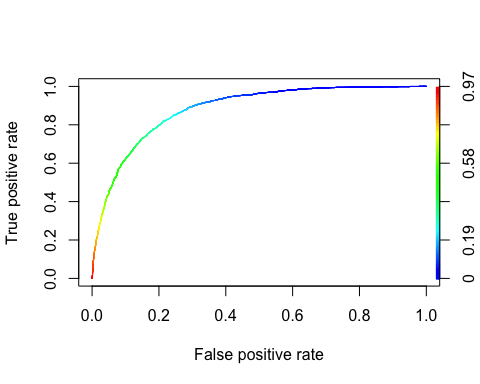Step 8) Improve the model

You can try to add non-linearity to the model with the interaction between

• age and hours.per.week
• gender and hours.per.week.

You need to use the score test to compare both model

formula_2 <- income~age: hours.per.week + gender: hours.per.week + . logit_2 <- glm(formula_2, data = data_train, family = ‘binomial’) predict_2 <- predict(logit_2, data_test, type = ‘response’) table_mat_2 <- table(data_test\$income, predict_2 > 0.5) precision_2 <- precision(table_mat_2) recall_2 <- recall(table_mat_2) f1_2 <- 2 * ((precision_2 * recall_2) / (precision_2 + recall_2)) f1_2

Output:

##  0.6109181

The score is slightly higher than the previous one. You can keep working on the data a try to beat the score.

## Summary

We can summarize the function to train a logistic regression in the table below:

Package Objective function argument
- Create train/test dataset create_train_set() data, size, train
glm Train a Generalized Linear Model glm() formula, data, family*
glm Summarize the model summary() fitted model
base Make prediction predict() fitted model, dataset, type = ‘response’
base Create a confusion matrix table() y, predict()
base Create accuracy score sum(diag(table())/sum(table()
ROCR Create ROC : Step 1 Create prediction prediction() predict(), y
ROCR Create ROC : Step 2 Create performance performance() prediction(), ‘tpr’, ‘fpr’
ROCR Create ROC : Step 3 Plot graph plot() performance()

The other GLM type of models are: